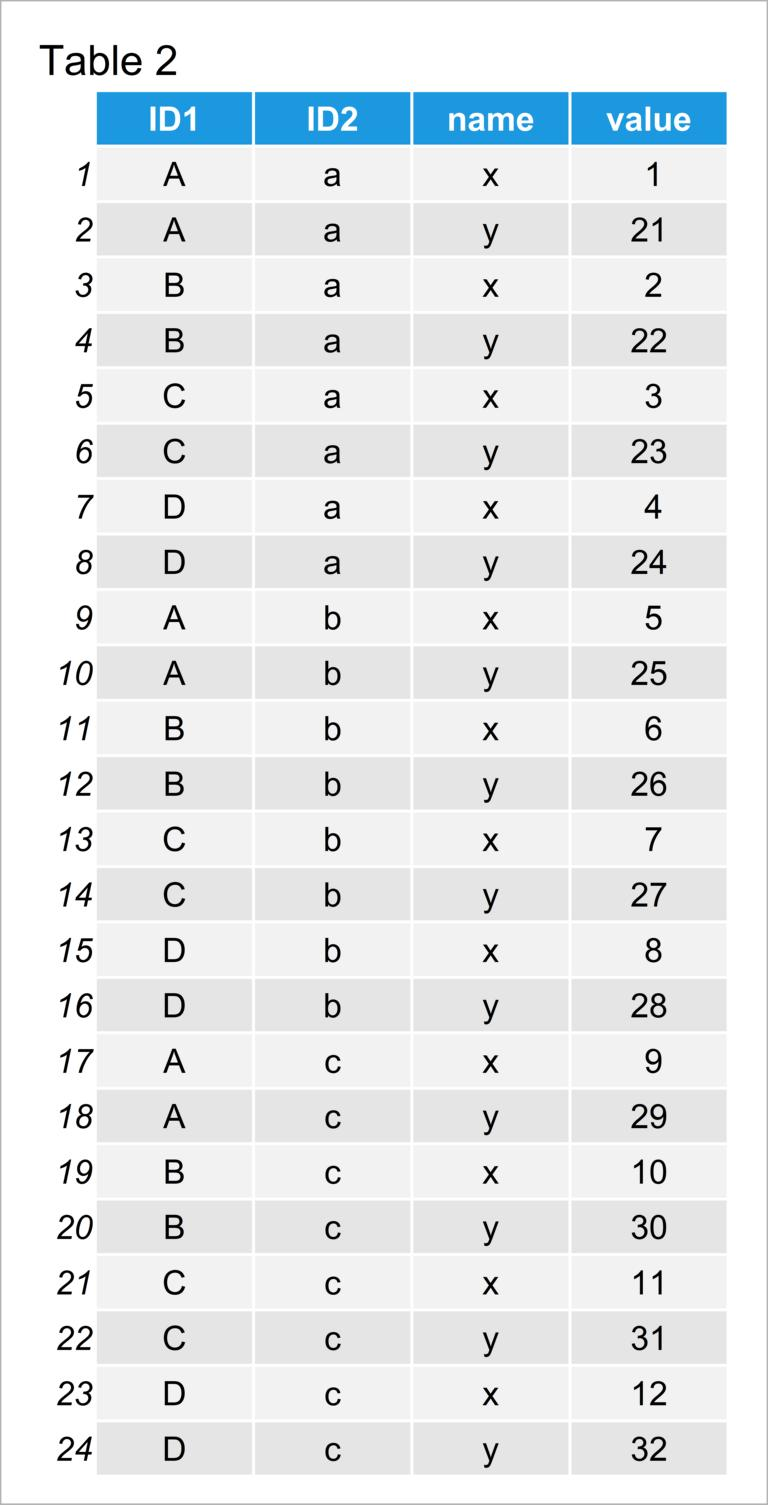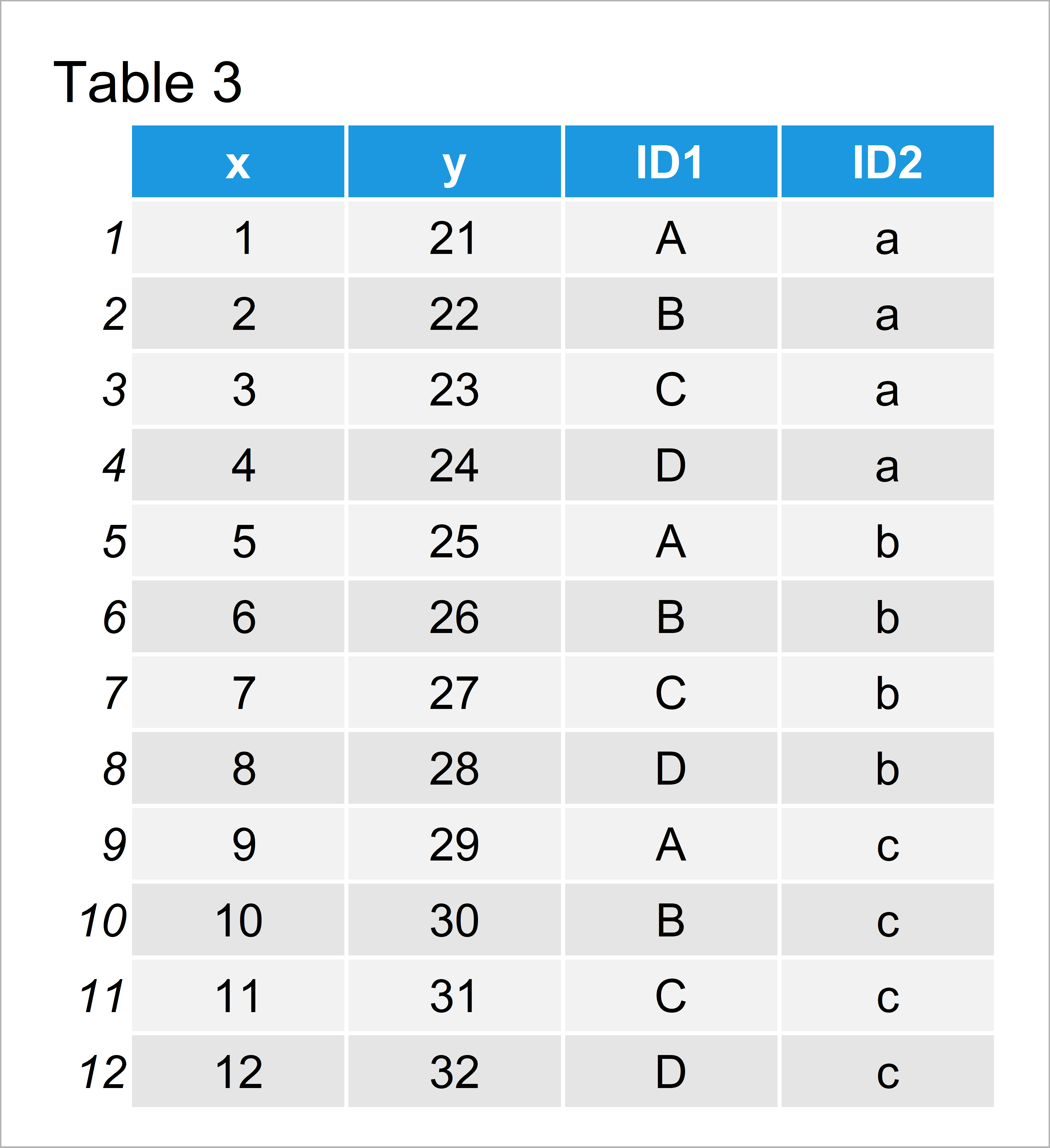# pivot_longer & pivot_wider Functions of tidyr Package in R (2 Examples)

In this R tutorial you’ll learn how to apply the pivot_longer and pivot_wider functions of the tidyr add-on package.

The content of the page looks like this:

Let’s start right away.

## Example Data & Add-On Packages

Consider the following example data:

```data <- data.frame(ID1 = LETTERS[1:4], # Create example data ID2 = rep(letters[1:3], each = 4), x = 1:12, y = 21:32) data # Print example data```Table 1 visualizes the output of the RStudio console returned by the previous R programming code and visualizes the structure of our example data – It is constituted of twelve rows and four columns.

The variables ID1 and ID2 contain id values that we will use to reshape our data frame. Note that our example data frame has a wide format.

For the examples of this tutorial, we also need to install and load the tidyr package:

```install.packages("tidyr") # Install & load tidyr package library("tidyr")```

Now, we are set up and can move on to the examples!

## Example 1: Convert Wide to Long Data Using pivot_longer() Function

Example 1 shows how to reshape our data frame from wide to long format using the pivot_longer function of the tidyr package.

For this, we have to specify the name of our data frame (i.e. data) and the columns that we want to pivot into longer format (i.e. x and y):

```data_long <- pivot_longer(data = data, # Convert wide to long cols = c("x", "y")) data_long # Print long data```In Table 2 it is shown that we have created a data set in long format by executing the previous R syntax.

Please note that the output of the pivot_longer function is a tibble, even though we have used a data frame as input. In case you prefer to work with data frames, you have to use the as.data.frame function to convert the tibble back to the data.frame class.

## Example 2: Convert Long to Wide Data Using pivot_wider() Function

This example shows how to pivot our long data set that we have created in Example 1 to a wide data format.

For this, we can use the pivot_wider function. Within the pivot_wider function, we only have to specify our long data as shown below:

```data_wide <- pivot_wider(data = data_long) # Convert long to wide data_wide # Print wide data```Table 3 shows the output of the previous code – A tibble in wide data format.

Note that the structure of Table 3 is exactly the same as Table 1 (i.e. our wide input data frame).

## Video & Further Resources

In case you need more info on the R syntax of this tutorial, you may want to watch the following video tutorial of my YouTube channel. I illustrate the R syntax of this article in the video:

Additionally, you may want to read some of the related articles on my website. I have released numerous tutorials already:

Summary: You have learned in this tutorial how to reshape data sets using pivot_longer and pivot_wider in the R programming language.

Note that pivot_longer and pivot_wider are also replacing older functions of the tidyverse such as gather and spread. So it’s definitely worth to add those functions to your repertoire!